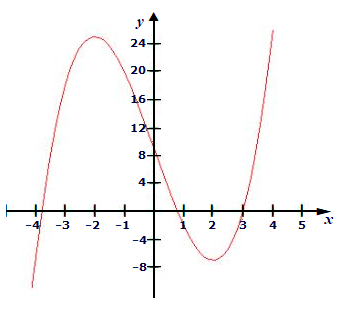# Given the graph of f(x), find the following: a) The interval(s) where the graph of f(x) is...

## Question:

Given the graph of {eq}f(x) {/eq}, find the following:

a) The interval(s) where the graph of {eq}f(x) {/eq} is increasing and decreasing

b) Any local extrema

c) Any absolute extrema## Graphs:

Answer to such types of question can be only given qualitatively with the available information. We have to carefully observe the intervals for the required answer and answer the questions.

## Answer and Explanation:### a) The interval(s) where the graph of {eq}f(x) {/eq} is increasing and decreasing :

From the graph we can see that :

Function is increasing in {eq}\boxed{[-4,-2 ) \cup (2,4 ]} {/eq}

Function is decreasing in {eq}\boxed{(-2,2)} {/eq}

### b) Any local extrema

From graph we can see local extrema at the point {eq}\boxed{x=-2,2} {/eq}

### c) Any absolute extrema

From graph we can see absolute extrema at the point {eq}\boxed{x=4} {/eq}

#### Learn more about this topic:Graphs: Types, Examples & Functions

from High School Algebra II: Help and Review

Chapter 16 / Lesson 11
355K